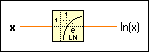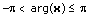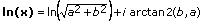# Natural Logarithm Function

## LabVIEW 2018 Help

Edition Date: March 2018
Part Number: 371361R-01
View Product InfoLabVIEW 2016 HelpLabVIEW 2017 HelpLabVIEW 2018 HelpLabVIEW 2019 HelpLabVIEW 2020 Help

Owning Palette: Exponential Functions

Requires: Base Development System

Computes the base e natural logarithm of x.

If x is 0, ln(x) is –. If x is not complex and is less than 0, ln(x) is NaN.Note  For very small values of x, the Natural Logarithm (Arg +1) function is more accurate than adding 1 to x then using this function.

The connector pane displays the default data types for this polymorphic function.x can be a scalar number, array or cluster of numbers, array of clusters of numbers, and so on.ln(x) is of the same numeric representation as x. When x is of the form x = a + bi, that is, when x is complex, the following equation defines the natural logarithm ln(x): ln(x) = ln(|x|) + i arg(x) where arg(x) is the phase of x over the interval. In other words, LabVIEW uses the following equation:## Natural Logarithm Details

When you wire matrix data as an input to this function, a VI that includes subVIs that work with the matrix data type replaces the function. The resulting VI has the same icon but contains a matrix-specific algorithm. The node remains a VI if you disconnect the matrix from the input(s). Wire other data types as inputs to restore the original function. If you wire a data type to a function and that data type causes a basic math operation to fail, the function returns an empty matrix or NaN.